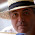## 11 August 2014

### Quiz 6: Print alphabet followed by number of times it appears continuously in a string.

Problem:
Print alphabet followed by number of times it appears continuously in a string

Sample Input:
aaabbccccddde

Sample Output:
a3b2c4d3e1

Solution:

my \$a = "aaabbccccddde";
my @arr = split("",\$a);
my \$tmp = 1;
for(my \$i=0;\$i<@arr;\$i++)
{
if(\$arr[\$i] eq \$arr[\$i+1])
{
\$tmp++;
}
else{
print "\$arr[\$i]\$tmp";
\$tmp = 1;
}
}

Tips:
• remember to set tmp variable as 1 whenever a new character is found ex char b after char a.

#### 3 comments:

1.my \$input = 'aaabbccccddde';

\$input =~ s/(([a-z])\2*)/\$2 . length(\$1)/ge;

print "\$input\n";

2.Another Method:
#!/usr/bin/perl -w

print "Please enter alphabet:";
@string = split(/\s+/,<>);

foreach \$alpha (@string)
{
\$count{\$alpha}++;
#print "\$alpha :\$count{\$alpha}\n";
}

@alphaRep = keys %count;

foreach \$beta(@alphaRep)
{
print "\$beta is came \$count{\$beta}times\n";
}

1.@santosh correction 1: split(//,<>)
correction 2, for aaaba, output should be a3b1a1, your solution gives b1a4